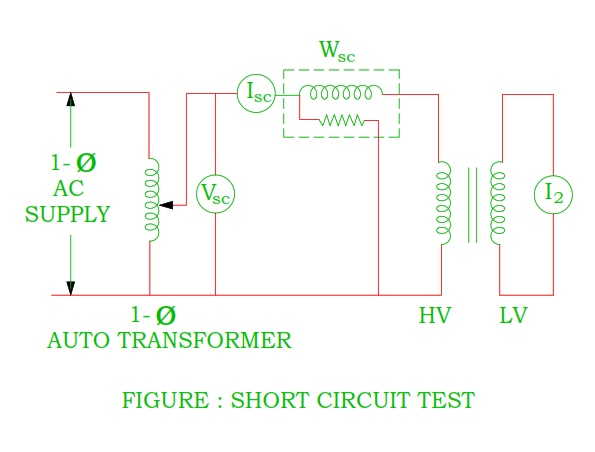## 17/01/2021

### To perform short circuit test on single phase transformer

Significance

The short circuit on the single phase transformer is performed in order to find out equivalent circuit parameters of the transformer. This test is performed to find out

• Equivalent impedance as referred to primary (Z01) or secondary side (Z02)
• Equivalent leakage reactance as referred to primary (X01)  or secondary side (X02)
• Equivalent resistance as referred to primary (R01)  or secondary side (R02)
• Voltage drop as referred to primary or secondary side
• Voltage regulation at any load and power factor
• Voltage transformation ratio

Instruments required

 Instrument Specification AC voltmeter AC ammeter Wattmeter Multi meter Single phase auto transformer

Theory

• In this test, the low voltage winding is usually short circuited by thick wire or ammeter and supply is given to the high voltage winding.
• The supply to the high voltage winding is given by using auto transformer.
• The applied voltage Vsc is gradually increases till the ammeter shows the full load current.
• As the applied voltage Vsc is very low ( only 5 – 10% of the normal voltage ), core flux is very small, iron losses are small so it is neglected.
•  The wattmeter Wsc shows full load copper losses of the transformer.
• Full load copper losses of both winding Wsc = Isc2 R01

⸫  R01 = Wsc / Isc2

• Equivalent impedance as referred to primary side

Zsc = Z01 = Vsc / Isc

⸫  X01 = √ ( Z012 – R012 )

• The voltage transformation ratio ( k ) can easily find out by taking reading of HV winding and LV winding.
• The transformer equivalent circuit parameter can easily determined as referred to secondary side after calculating voltage transformation ratio ( k ).

R02 = R01 / k2

X02 = X01 / k2

Z02 = Z01 / k2

Circuit diagramProcedure

• Make connection as per circuit diagram
• Keep the voltage of the auto transformer with zero voltage position
• Switch on the supply and slowly increase the auto transformer voltage until the ammeter shows the full load current
• Note down the reading of ammeter, voltmeter and wattmeter.

Precaution

• It should be kept in mind that setting of the auto transformer must be in minimum or zero voltage position before switch on the supply.

Observation table

 Short circuit voltage Short circuit current Short circuit power Vsc Isc Wsc

Calculation

 Short circuit input Power Wsc = Vsc Isc Cos Φsc Short circuit power factor & power factor angle Cos Φsc = Wsc / Vsc Isc    Φsc = Cos – 1( Wsc / Vsc Isc ) Transformer winding resistance as referred to primary side R01 = Wsc / Isc2 Transformer winding impedance as referred to primary side Vsc / Isc Transformer winding reactance as referred to primary side X01 = √ ( Z012 – R012 ) Voltage transformation ratio ( k ) k = I1 / I2 = Isc / I2 Transformer winding resistance as referred to secondary side R02 = R01 / k2 Transformer winding impedance as referred to secondary side Z02 = Z01 / k2 Transformer winding reactance as referred to secondary side X02 = X01 / k2 Full load copper losses Isc2 R01 Voltage drop as referred to primary side Isc ( R01 + j X01 ) Voltage drop as referred to secondary side I2 ( R02 + j X02 ) Voltage regulation at any load and any power factor + sign for lagging power factor – sign for leading power factor 0V2 = No load voltage I1 R01 Cos Ф2 ± I1 X01 Sin Ф2 / ( 0V2 )                    OR I2 R02 Cos Ф2 ± I2 X02 Sin Ф2 / ( 0V2 )

Conclusion

• The wattmeter shows full load copper losses and iron losses of the transformer.
• As the supply voltage is only small amount of rated voltage, iron losses is neglected therefore the wattmeter shows full load copper losses.

Which winding of the transformer is short circuited? LV winding or HV winding. Give reason

• The LV winding of the transformer is short circuited.
• Let us try to understand reason for that, consider a 10 kVA, 1100 V / 220 V transformer, if supply is given to LV side, voltage required for full load current flows through winding during short circuit of HV winding lies between 5% to 10% of rated value i.e ( 220 × 5 ) / 100 = 11 V to ( 220 × 10 ) / 100 = 22 V which is very small.
• At low voltage ( 11 V to 22 V ), high precision reading would not be obtained by ordinary meter.

You may also like :